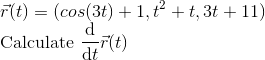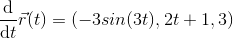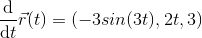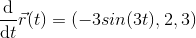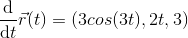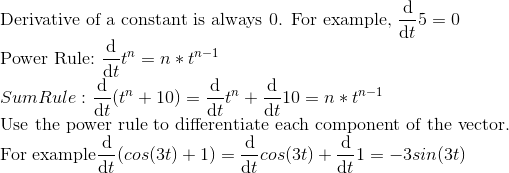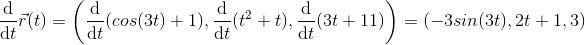# Calculus 2 : Derivatives of Vectors

## Example Questions

### Example Question #11 : Derivatives Of Vectors

We define the vector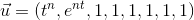.

What is the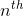derivative of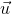?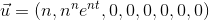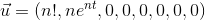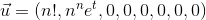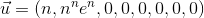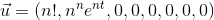Explanation:

To obtain the derivative we need to compute the derivative of each component. Since we are looking for the derivative of order n we have to differentiate each component n times to obtain the required result.

We know that :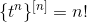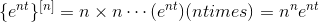The nth derivatives of the constant is 0.

Thereore we obtain: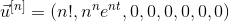### Example Question #12 : Derivatives Of Vectors

Let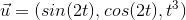.

What is the third derivative of?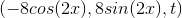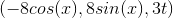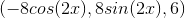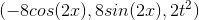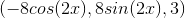Explanation:

To obtain the derivative, we simply differentiate 3 times each of the components.

Using the Chain Rule and the Power Rule we have :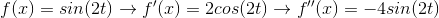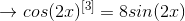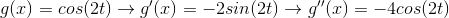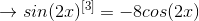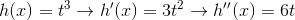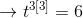### Example Question #13 : Derivatives Of Vectors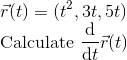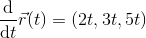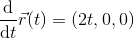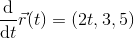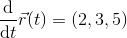Explanation: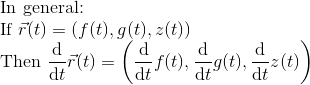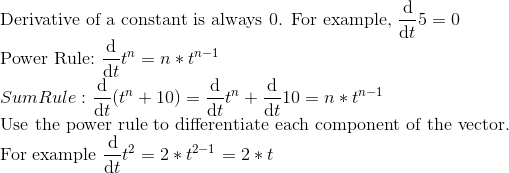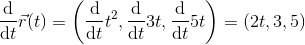### Example Question #14 : Derivatives Of Vectors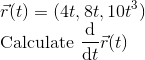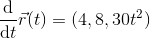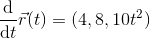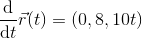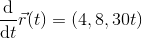Explanation: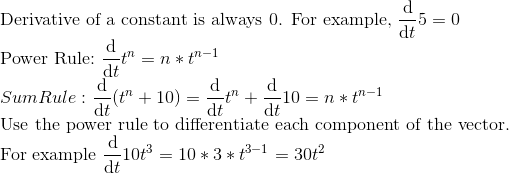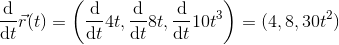### Example Question #15 : Derivatives Of Vectors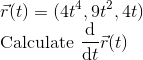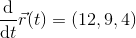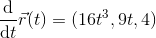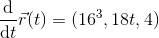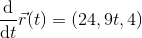Explanation: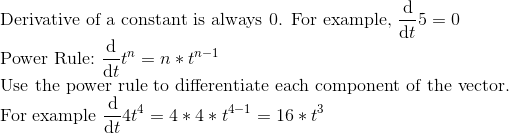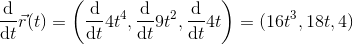### Example Question #16 : Derivatives Of Vectors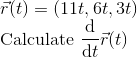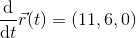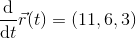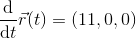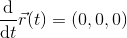Explanation: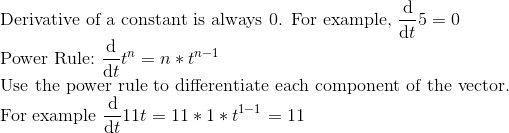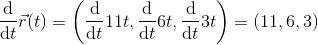### Example Question #17 : Derivatives Of Vectors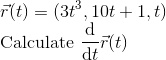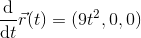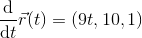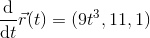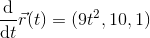Explanation: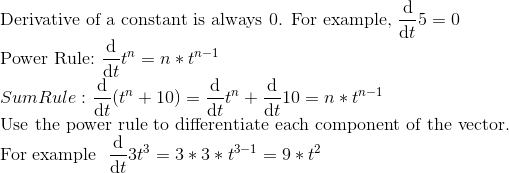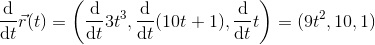### Example Question #141 : Vector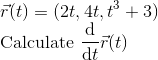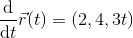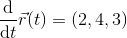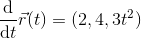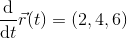Explanation: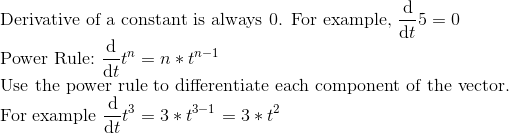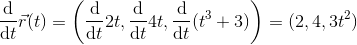### Example Question #142 : Vector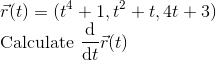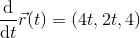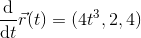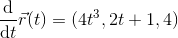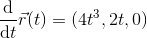Explanation: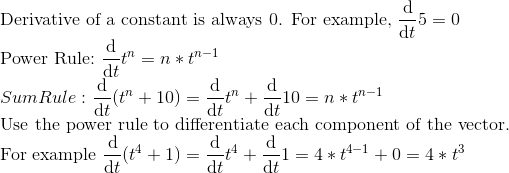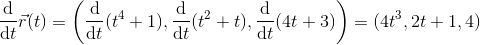### Example Question #143 : Vector